# Analysis of order statistics of uncertain variables

## Abstract

In this paper, a concept of order uncertain variable is introduced as a main tool in analysis of k-out-of-n system with uncertain lifetimes. Then, by the operational law of uncertain variable, the uncertainty distribution of order uncertain variable is deduced. At last, the k-out-of-n system is studied, and the uncertainty distribution of k-out-of-n system lifetime is given.

## Introduction

The real world is filled with indeterminacy. In fact, randomness and fuzziness are two cases of indeterminacy, behind which probability theory and credibility theory have been proposed. However, some practical systems behave neither random nor fuzzy, so it cannot be modeled precisely by probability theory or credibility theory. In order to deal with this type of uncertainty, Liu  founded an uncertainty theory in 2007, which is a branch of mathematics based on normality, duality, subadditivity, and product axioms. Since then, based on uncertainty theory, significant work both in theory and engineering has been done by many researchers. As an application of uncertainty theory, Liu  proposed a spectrum of uncertain programming which is a type of mathematical programming involving uncertain variables and applied uncertain programming to system reliability design, facility location problem, vehicle routing problem, project scheduling problem, finance, and so on. In addition, Li and Liu  presented uncertain logic in which the truth value is defined as the uncertain measure that the proposition is true. Furthermore, uncertain inference was pioneered by Liu  as a process of deriving consequences from uncertain knowledge or evidence via the tool of conditional uncertainty. Other researchers also have done a lot of theoretical work in uncertainty theory, such as Chen , Chen and Liu , Gao , Gao et al. , Liu , Peng , Peng and Chen , Qin and Kar , Wang and Peng , Yang , Yao , You  and Zhu , etc.

Problems of k-out-of-n system play an important role in system reliability design. Up to now, k-out-of-n system with random lifetimes has been researched in many books related to system reliability design. This paper aims to give some analysis of k-out-of-n system with uncertain lifetimes. Based on the uncertainty theory, this paper gives a new concept of order uncertain variable, which is used as a tool to analyze k-out-of-n system with uncertain lifetimes in this paper. It is similar to the order statistics (Balakrishnan and Cohen ) in mathematical statistics. However, order uncertain variable just requires that the initial uncertain variables are independent. Besides, the uncertainty distribution of order statistics of uncertain variables has a much simpler form than the ones of random variables.

The remainder of this paper is organized as follows. In Section “Preliminary”, basic concepts and properties regarding uncertain variables are reviewed. In Section “Order uncertain variable”, the concept of order uncertain variable is given. In Section “Uncertainty distribution of order uncertain variable”, the uncertainty distribution of order uncertain variable is deduced. In Section “k-out-of-n system with uncertain lifetimes”, the order uncertain variable is used as a tool to analyze k-out-of-n system with uncertain lifetimes.

## Preliminary

In this section, we introduce some foundational concepts and properties of uncertainty theory, which is used throughout this paper.

Let Γ be a nonempty set, anda σ-algebra over Γ. Each elementis assigned a number. In order to ensure that the numberhas certain mathematical properties, Liu  presented the following four axioms:

### Axiom1.

(Normality Axiom)### Axiom2.

(Duality Axiom)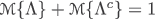for any event Λ.

### Axiom3.

(Subadditivity Axiom) For every countable sequence of events {Λ i }, we have### Definition1.

(Liu ) The set functionis called an uncertain measure if it satisfies the normality, duality, and subadditivity axioms.

The triplet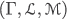is called an uncertainty space. Furthermore, the product uncertain measure on the product σ-algebrais defined by Liu  as follows:

### Axiom4.

(Product Axiom) Letbe uncertainty spaces for i=1,2, The product uncertain measureis an uncertain measure satisfying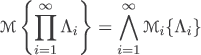where Λ i are arbitrarily chosen events fromfor i=1,2,, respectively.

### Definition2.

(Liu ) An uncertain variable is a measurable function ξ from an uncertainty spaceto the set of real numbers, i.e., for any Borel set B of real numbers, the set

$$\{\xi\in B\}=\{\gamma\in \Gamma\mid \xi(\gamma)\in B\}$$

is an event.

### Definition3.

(Liu ) The uncertainty distribution Φ:→[0,1] of an uncertain variable ξ is defined by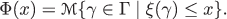### Definition4.

(Liu ) The uncertain variables ξ 1,ξ 2,,ξ n are said to be independent if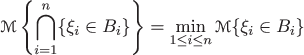for any Borel sets B 1,B 2,,B n of real numbers.

More generally, the independence of uncertain variables was given by Liu .

### Theorem1.

(Liu ) The uncertain variables ξ 1,ξ 2,,ξ n are said to be independent if and only if### Definition5.

(Liu ) Let ξ be an uncertain variable. Then, the expected value of ξ is defined by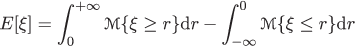provided that at least one of the two integrals is finite.

From Remark 1.6 in Liu (), the following theorem is given without proof.

### Theorem2.

(Liu ) Let ξ 1,ξ 2,,ξ n be independent uncertain variables, and $$f : \mathfrak {R}^{n} \rightarrow \mathfrak {R}$$ a measurable function. Then, ξ=f(ξ 1,ξ 2,,ξ n ) is an uncertain variable such that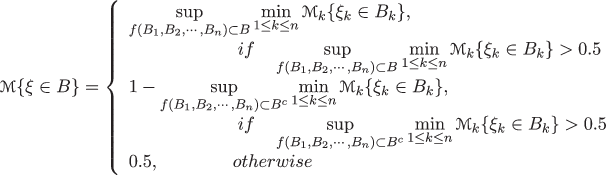where B,B 1,B 2,,B n are Borel sets of real numbers.

### Lemma1.

Let ξ 1,ξ 2,,ξ n be independent uncertain variables taking values in {0,1}, such thatwhere 0≤a i ≤1,i=1,2,,n, and a 0a 1a n a n+1=1. Then, for any $$0\leq k\leq n,k\in \mathbb {N}$$, we have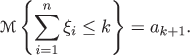### Proof.

Because ξ i takes values in {0,1}, i=1,2,,n, according to Equation ??, we can getIt is easy to verify that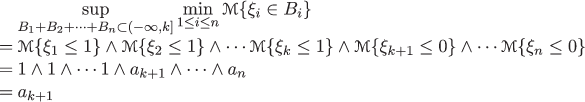andThen, we get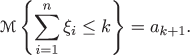## Order uncertain variable

### Definition6.

Let f i :n,i=1,2,,n be a series of measurable functions. We call f 1,f 2,,f n the order functions of n if for any (x 1,x 2,,x n )n,

$$f_{i}(x_{1},x_{2},\cdots,x_{n})=x^{(i)},\quad i=1,2,\cdots,n$$

where {x (1),x (2),,x (n)} is the the rearrangement of {x 1,x 2,,x n } in ascending order of magnitude, that is, x (1)x (2)x (n).

### Definition7.

Let ξ 1,ξ 2,,ξ n be independent uncertain variables, and f 1,f 2,,f n order functions of n. Define

$$\xi^{(i)}=f_{i}(\xi_{1}, \xi_{2}, \cdots, \xi_{n}),\quad i=1,2,\cdots,n.$$

Then, ξ (1),ξ (2),,ξ (n) are called order uncertain variables of ξ 1,ξ 2,,ξ n , and each ξ (i) is called the ith order uncertain variable of ξ 1,ξ 2,,ξ n .

To be more explicit, consider the case when n=2 and the relationship between ξ i and ξ (i)(i=1,2) is

$$\begin{array}{lllll} \xi^{(1)}=\xi_{1} &and &\xi^{(2)}=\xi_{2} & when &\xi_{1} < \xi_{2},\\ \xi^{(1)}=\xi_{2} &and &\xi^{(2)}=\xi_{1} & when &\xi_{2} < \xi_{1}. \end{array}$$

Similarly, for n=3 the relationship among the respective uncertain variables is

$$\begin{array}{llllll} \xi^{(1)}=\xi_{1}, &\xi^{(2)}=\xi_{2}, &and &\xi^{(3)}=\xi_{3} &when &\xi_{1} < \xi_{2} < \xi_{3},\\ \xi^{(1)}=\xi_{1}, &\xi^{(2)}=\xi_{3}, &and &\xi^{(3)}=\xi_{2} &when &\xi_{1} < \xi_{3} < \xi_{2},\\ \cdots\\ \xi^{(1)}=\xi_{3}, &\xi^{(2)}=\xi_{2}, &and &\xi^{(3)}=\xi_{1} &when & \xi_{3} < \xi_{2} < \xi_{1}. \end{array}$$

The most frequently encountered functions of order uncertain variable are ξ (1) and ξ (n). Obviously, $$\xi ^{(1)}=\min \limits _{1\leq k\leq n} \xi _{k}$$, and $$\xi ^{(n)}=\max \limits _{1\leq k\leq n} \xi _{k}$$.

## Uncertainty distribution of order uncertain variable

In this section, the uncertainty distribution of the kth order uncertain variable for k=1,2,,n will be given.

### Theorem3.

Let ξ 1,ξ 2,,ξ n be independent uncertain variables with distributions Φ 1(x),Φ 2(x),,Φ n (x), respectively. The uncertainty distribution of the kth (k=1,2,,n) order uncertain variable ξ (k) is

$$\Psi_{k}(x)=f_{n-k+1}(\Phi_{1}(x),\Phi_{2}(x),\cdots,\Phi_{n}(x))$$
((1))

where f k :n,k=1,2,,n are order functions of n.

### Proof.

For any fixed x, define a series of new variables

$$\eta_{i}(x)=\left\{ \begin{array}{lll} 0,& if &\xi_{i}\leq x\\ 1, & if &\xi_{i}>x \end{array} \right.$$

where i=1,2,,n. Then, η 1(x),η 2(x),,η n (x) are independent, and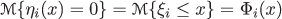for each i. According to the definitions of uncertain variable and order uncertain variable, by Lemma 1, we have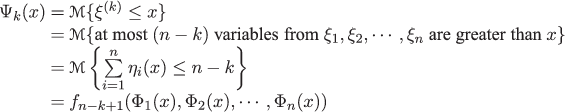where f k :n,k=1,2,,n are order functions of n. The theorem is proved. □

### Corollary1.

Let ξ 1,ξ 2,,ξ n be independent uncertain variables with the same uncertainty distribution Φ(x), and let ξ (1),ξ (2),,ξ (n) be the corresponding order uncertain variables. Then, for any k=1,2,,n, the kth order uncertain variable has uncertainty distribution Φ(x).

### Proof.

It follows from Theorem 2 immediately. □

### Corollary2.

Let ξ 1,ξ 2,,ξ n be independent uncertain variables with the same uncertainty distribution Φ(x), and let ξ (1),ξ (2),,ξ (n) be the corresponding order uncertain variables. Then, for any k=1,2,,n, the kth order uncertain variable has expected value E[ξ 1] provided that E[ξ 1] exists.

### Proof.

It follows from Corollary 1 and Definition 4 immediately. □

## k-out-of-n system with uncertain lifetimes

System reliability design with uncertain lifetimes was first studied by Liu . The k-out-of-n system is a significant kind of problem in system reliability design. In this section, order uncertain variable is used as a tool in analysis of k-out-of-n system with uncertain lifetimes.

A system of n components is called a k-out-of-n system if it remains operational only if at least k components continue to function. Assume that the ith element of this component has independent lifetime ξ i with uncertainty distribution Φ i (x), i=1,2,,n, respectively. Then, what is the distribution Φ(x) of this system lifetime η?

Let ξ (1),ξ (2),,ξ (n) be the order uncertain variables of ξ 1,ξ 2,,ξ n . Then, for any x>0,x, by Theorem 2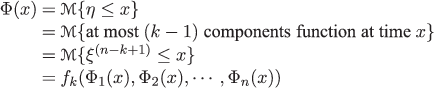where f k :n,k=1,2,,n are order functions of n. Thus, we get the uncertainty distribution of η.

The special cases k=1 and k=n correspond respectively to parallel system and series system (Figure 1). According to the formula (Equation ??), the uncertainty distribution Φ p (x) of parallel system lifetime η p is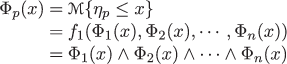and the uncertainty distribution Φ s (x) of series system lifetime η s is## Conclusion

In order to analyze k-out-of-n system with uncertain lifetimes, we presented a new concept of order uncertain variable and gave uncertainty distribution of order uncertain variable in this paper. By using order uncertain variable, the uncertainty distribution of k-out-of-n system lifetime was deduced.

## References

1. Chen, X, Dai, W: Maximum entropy principle for uncertain variables. Int. J. Fuzzy Syst. 13(3), 232–236 (2011).

2. Chen, X, Liu, B: Existence and uniqueness theorem for uncertain differential equations. Fuzzy Optimization Decis. Making. 9(1), 69–81 (2010).

3. Gao, X: Some properties of continuous uncertain measure. Int. J. Uncertainty Fuzziness Knowledge-Based Syst. 17(3), 419–426 (2009).

4. Gao, Y, Yang, L, Li, S, Kar, S: On distribution function of the diameter in uncertain graph. Inf. Sci. 296, 61–74 (2015).

5. Li, X, Liu, B: Hybrid logic and uncertain logic. J. Uncertain Syst. 3(2), 83–94 (2009).

6. Liu, B: Uncertainty Theory. 2nd ed. Springer-Verlag, Berlin (2007).

7. Liu, B: Theory and Practice of Uncertain Programming. 2nd ed. Springer-Verlag, Berlin (2009).

8. Liu, B: Some research problem in uncertainty theory. J. Uncertain Syst. 3(1), 3–10 (2009).

9. Liu, B: Polyrectangular theorem and independence of uncertain vectors. J. Uncertainty Anal. Appl. 1, 9 (2013). doi:10.1186/2195-5468-1-9.

10. Balakrishnan, N, Cohen, AC: Order statistics and inference: estimation methods. Academic Press, Inc., San Diego (1991). ISBN 0120769484, 9780120769483.

11. Peng, Z, Iwamura, K: Some properties of product uncertain measure. J. Uncertain Syst. 6(4), 263–269 (2012).

12. Peng, Z, Chen, X: Uncertain systems are universal approximators. J. Uncertainty Anal. Appl. 2, 13 (2014). doi:10.1186/2195-5468-2-13.

13. Qin, Z, Kar, S: Single-period inventory problem under uncertain environment. Appl. Math. Comput. 219(18), 9630–9638 (2013).

14. Wang, X, Peng, Z: Method of moments for estimating uncertainty distributions. J. Uncertainty Anal. Appl. 2, 5 (2014). doi:10.1186/2195-5468-2-5.

15. Yang, L, Liu, P, Li, S, Gao, Y, Ralescu, D: Reduction methods of type-2 uncertain variables and their applications to solid transportation problem. Inf. Sci. 291, 204–237 (2015).

16. Yao, K: Extreme values and integral of solution of uncertain differencial equation. J. Uncertainty Anal. Appl. 1, Article, 2 (2013).

17. You, C: Some convergence theorems of uncertain sequences. Math. Comput. Modell. 49(3-4), 482–487 (2009).

18. Zhu, Y: Uncertain optimal control with application to a portfolio selection model. Cybernet. Syst. 41(7), 535–547 (2010).

## Acknowledgments

The research was supported by the Fundamental Research Funds for the central universities (No. 2014JBM155) and the State Key Laboratory of Rail Traffic Control and Safety (No. RCS2014ZQ001 and No. RCS2014ZT22, Beijing Jiaotong University).

## Author information

Authors

### Corresponding author

Correspondence to Rong Gao.

## Rights and permissions